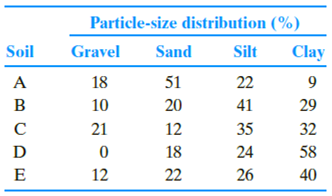Chapter 5, Problem 5.2PPrinciples of Geotechnical Enginee...

9th Edition
Braja M. Das + 1 other
ISBN: 9781305970939

Solutions

Chapter
SectionPrinciples of Geotechnical Enginee...

9th Edition
Braja M. Das + 1 other
ISBN: 9781305970939
Textbook Problem

The gravel, sand, silt and clay contents of five different soils are given below. Classify the soils using the U.S. Department of Agriculture textural classification chart.To determine

The classification of soil based on United States department of agriculture textural classification chart.

Explanation

Calculation:

Consider soil A:

Determine the modified percentage of sand using the formula.

Modifiedpercentageofsand=Percentageofsand100Percentageofgravel

Substitute 51 % for percentage of sand and 18 % for percentage of gravel.

Modifiedpercentageofsand=5110018×100=62.2%

Determine the modified percentage of silt using the formula.

Modifiedpercentageofsilt=Percentageofsilt100Percentageofgravel

Substitute 22 % for percentage of silt and 18 % for percentage of gravel.

Modifiedpercentageofsilt=2210018×100=26.8%

Determine the modified percentage of clay using the formula.

Modifiedpercentageofclay=Percentageofclay100Percentageofgravel

Substitute 9 % for percentage of clay and 18 % for percentage of gravel.

Modifiedpercentageofclay=910018×100=10.9%

Refer Figure (5.1) “U.S. Department of agriculture textural classification (USDA)”

in the text book.

Mark the percentage of sand for soil A as 62.2 %, percentage of silt as 26.8 %, and percentage of clay as 10.9 % in Figure (5.1) and mark the point of intersection of three lines.

Show the percentage of sand, silt, and clay of soil A as in Figure (1).

Refer Figure (1).

Hence, the classification of soil A as per the United States department of agriculture textural classification chart is Sandyloam_.

Consider soil B:

Determine the modified percentage of sand using the formula.

Modifiedpercentageofsand=Percentageofsand100Percentageofgravel

Substitute 20 % for percentage of sand and 10 % for percentage of gravel.

Modifiedpercentageofsand=2010010×100=22.2%

Determine the modified percentage of silt using the formula.

Modifiedpercentageofsilt=Percentageofsilt100Percentageofgravel

Substitute 41 % for percentage of silt and 10 % for percentage of gravel.

Modifiedpercentageofsilt=4110010×100=45.6%

Determine the modified percentage of clay using the formula.

Modifiedpercentageofclay=Percentageofclay100Percentageofgravel

Substitute 29 % for percentage of clay and 10 % for percentage of gravel.

Modifiedpercentageofclay=2910010×100=32.2%

Mark the percentage of sand for soil B as 22.2 %, percentage of silt as 45.5 %, and percentage of clay as 32.2 % in Figure (5.1) and mark the point of intersection of three lines.

Show the percentage of sand, silt, and clay of soil B as in Figure (2).

Refer Figure (2).

Thus, classification of soil B as per the United States department of agriculture textural classification chart is Clayloam_.

Consider soil C:

Determine the modified percentage of sand using the formula.

Modifiedpercentageofsand=Percentageofsand100Percentageofgravel

Substitute 12 % for percentage of sand and 21 % for percentage of gravel.

Modifiedpercentageofsand=1210021×100=15.2%

Determine the modified percentage of silt using the formula.

Modifiedpercentageofsilt=Percentageofsilt100Percentageofgravel

Substitute 35 % for percentage of silt and 21 % for percentage of gravel.

Modifiedpercentageofsilt=3510021×100=44

Still sussing out bartleby?

Check out a sample textbook solution.

See a sample solution

The Solution to Your Study Problems

Bartleby provides explanations to thousands of textbook problems written by our experts, many with advanced degrees!

Get Started

What percentage of the U.S. electric power is generated using renewable sources?

Engineering Fundamentals: An Introduction to Engineering (MindTap Course List)

Define supply chain management (SCM).

Principles of Information Systems (MindTap Course List)

List and define the common attributes of tasks within a WBS.

Principles of Information Security (MindTap Course List)

What are the three form factors for servers?

Enhanced Discovering Computers 2017 (Shelly Cashman Series) (MindTap Course List)

Define the term equal-pressure torch. How does it work?

Welding: Principles and Applications (MindTap Course List)